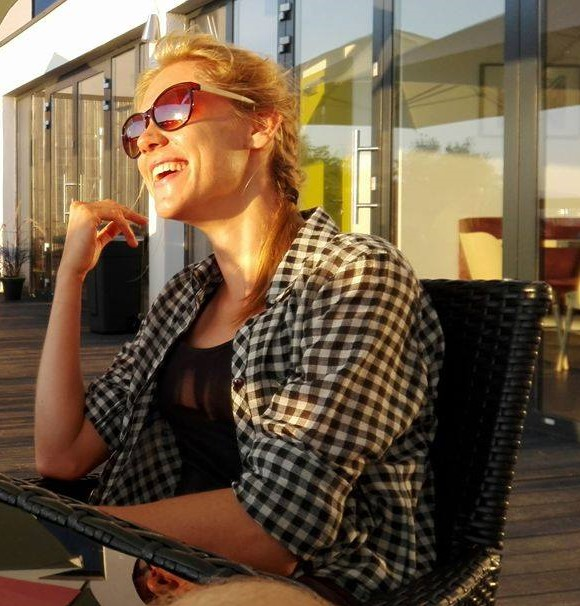# Approximate solutions and numerical analysis of a spring-mass running modelMy name is Zosia Wróblewska and I have just got a master’s degree in Applied Mathematics at Wrocław University of Science and Technology. My speciality is Mathematics for Industry and Commerce. During my studies I had a one semester exchange in Portugal at Técnico Lisboa as an Erasmus student. I chose this field of study, because the thing that interested me the most in mathematics, is the ability to describe and investigate reality. In particular, I became interested in topics related to biology. I already had an opportunity to test myself in issues that deal with the mechanics of the human body.

After graduating, I felt that I still needed to learn a lot, so I decided to start my PhD in biomechanics at the same faculty. In addition, I am currently studying at the University School of Physical Education in Wrocław, at athlete trainer speciality. I also train sprinting.

I am a person who is into new challenges and is really happy to cooperate and learn from others. That is why during my studies I participated in a few foreign mathematics programs. One of those was 33rd edition of the ECMI Modelling Week which took place in Darmstadt, Germany. During this event, we were working in small, international groups (under a supervision of the instructor) on projects based on the real-life problems. Our task was to predict water consumption by households, based on earlier meter readings. We received real data collected recurrently from big villas with gardens in Portugal. To solve this problems the knowledge gained during mathematical studies on such courses as time series was needed. We also learned a lot from our supervisor and from ourselves.

Moreover, at the turn of August and September this year, I participated in Applied Mathematics Summer School organized by King Abdullah University of Science and Technology (KAUST) in the Kingdom of Saudi Arabia. Here, together with other students, we proposed a price-formation model, with a population consisting of a finite number of agents storing and trading a commodity. I believe that participating in such projects and seeing how one can use (sometimes quite abstract) university knowledge to overcome industrial problems can be highly motivating for the future studies.

My master’s thesis, written under the supervision of Professor Łukasz Płociniczak, concerned modelling human movement. We consider the classic spring-mass model of running which is built upon an inverted elastic pendulum (cf. [1-3]).  In a natural way, during the contact phase, we get the governing system of two nonlinear ordinary differential equations:$\left[\begin{array}{c} L^2\ddot{\theta} + 2L\dot{L}\dot{\theta}\\ \ddot{L} \end{array}\right] = \left[\begin{array}{c} L\sin\theta\\ L\dot{\theta}^2+K(1-L)-\cos\theta \end{array}\right],$

where$L$ and$\theta$ are the polar coordinates of the mass originating at the foot point in stance (see Figure 1). Moreover, the nondimensional parameter$K$ is the spring stiffness.Figure 1. Schematic of the main model

The initial conditions for the polar coordinate system have the form:$\theta(0) = -\alpha, \quad \dot{\theta}(0) = \theta_d, \quad L(0) = 1, \quad \dot{L}(0) = - L_d,$

with$\theta_d = V_X \cos\alpha-V_Y \sin\alpha, \quad L_d = V_X \sin\alpha + V_Y \cos\alpha,$

where$V_X$ and$V_Y$ are the horizontal and vertical Froude numbers. Since the inverted elastic pendulum models the forwardly hoping leg we are faced with a peculiar boundary value problem to solve.

Problem. Let$(\theta(T,K),L(T,K))$ be the solution of the system with the above initial conditions. Find$K^*$ and the smallest time$T^*>0$ satisfying$\theta(T^*,K^*)= \alpha, \quad L(T^*,K^*) = 1.$

This means that the spring stiffness$K$ has to be determined to ensure that during the first cycle the spring will return to the equilibrium length precisely at the time for which the pendulum travels to the angle$\alpha$. The Problem can easily be solved numerically using the shooting method.

Next, we derive an approximate solution assuming a small angle of attack and a small spring compression:$|\theta| \ll 1$,$|\dot\theta| \ll 1$,$L \approx 1$. Then we get approximations for$\theta$ and$L$ in the following form:$\left[\begin{array}{c} \widetilde\theta(T)\\ \widetilde{L}(T) \end{array}\right] = \left[\begin{array}{c} \theta_d\sinh{T} - \alpha\cosh{T}\\ 1-{\omega}^{-1}L_d\sin{\omega T}- {\omega^{-2}}(1 - \cos{\omega T}) \end{array}\right],$

where$\omega^2= K$. Moreover, one of the theorems of the thesis shows that$K^* \approx \widetilde{K}^* := \left(\frac{\pi \theta_d}{2\alpha}\right)^2 = \left(\frac{\pi }{2\alpha}\left(V_X\cos\alpha-V_Y\sin\alpha\right)\right)^2,$

where$K^*$ is the solution to the Problem.Figure 2. Comparison between the numerically calculated value of$K^*$ and its approximation$\widetilde{K}^*$ calculated from the above formula for varying$\alpha$. Here$V_X=1$ and$V_Y=0.1$.

Numerical calculations showed very good compatibility of the approximation with the exact solution (see Figure 2). In addition, measurements of the running parameters of professional athletes allowed to calibrate the model.

The studied problem turned out to be interesting enough that together with Professor Płociniczak we wrote a paper on the analysis of equations related to the system with the use of Poincaré – Lindstedt series, see . A perturbative expansion with respect to the large spring stiffness is relevant for the solution of the Problem. Moreover, we used the obtained asymptotic estimates to prove that there exists a unique solution to the aforementioned boundary value problem and provided the same approximation to the sought stiffness.

 Blickhan, R. (1989).  The spring-mass model for running and hopping. Journal of Biomechanics, 22, 1217-1227.

 Geyer, H., Seyfarth, A. & Blickhan, R. (2005). Spring-mass running: simple approximate solution and application to gait stability. Journal of Theoretical Biology, 232, 315-328.

 McMahon, T. A. & Cheng, G. C. (1990). The mechanics of running: How does stiffness couple with speed? Journal of Biomechanics, 23, 65-78.

 Płociniczak, Ł. & Wróblewska, Z. (2019). Solution and asymptotic analysis of a boundary value problem in the spring-mass model of running. Online available at https://arxiv.org/abs/1903.02247(pdf).

By Zofia Wróblewska, student, Faculty of Pure and Applied Mathematics, Wrocław University of Science and Technology